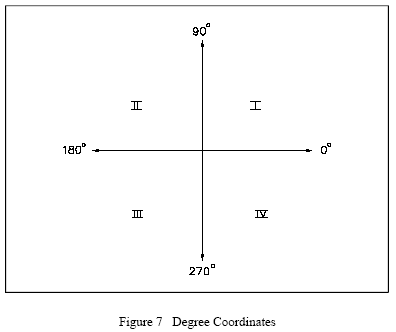Related Resources: physics

### Vector Identification - Physics

In textbooks, vector quantities are often represented by simply using a boldfaced letter (A, B, C, R). Particular quantities are predefined (F - force, V - velocity, and A - acceleration). Vector quantities are sometimes represented by. Regardless of the convention used, specific vector oquantities must include magnitude and direction (for example, 50 mph due north, or 50 lbf at 90°).

Vector quantities are graphically represented using the rectangular coordinate system, a two-dimensional system that uses an x-axis and a y-axis. The x-axis is a horizonal straight line. The y-axis is a vertical straight line, perpendicular to the x-axis. An example of a rectangular system is shown in Figure 3.The intersection of the axes is called the point of origin. Each axis is marked off in equal divisions in all four directions from the point of origin. On the horizonal axis (x), values to the right of the origin are positive (+). Values to the left of the origin are negative (-). On the vertical axis (y), values above the point of origin are positive (+). Values below the origin are negative (-). It is very important to use the same units (divisions) on both axes.

The rectangular coordinate system creates four infinite quadrants. Quadrant I is located above and to the right of the origin. Quadrant II is located above and to the left of the origin. Quadrant III is situated to the left and below the origin, and quadrant IV is located below and to the right of the origin (see Figure 3).

Graphic Representation of Vectors

With the coordinate system defined, the following explanation will illustrate how to locate vectors in that system.

First, using a ruler and graph paper, a rectangular coordinate system is laid out as described in the previous section. The x- and y-axes are labeled. Equal divisions are marked off in all four directions. Those to the right and above the point of origin are labeled positive (+). Those to the left and below the point of origin are labeled negative (-).

Beginning at the point of origin (intersection of the axes), a line segment of the proper length is shown along the x-axis, in the positive direction. This line segment represents the vector magnitude, or displacement. An arrow is placed at the "head" of the vector to indicate direction. The "tail" of the vector is located at the point of origin (see Figure 4).When vectors are drawn that do not fall on the x- or y-axes, the tail is located at the point of origin. Depending on the vector description, there are two methods of locating the head of the vector. If coordinates (x,y) are given, these values can be plotted to locate the vector head. If the vector is described in degrees, the line segment can be rotated counterclockwise from the x-axis to the proper orientation, as shown in Figure 5.Because the x- and y-axes define direction, conventional directional coordinates and degrees may also be used to identify the x- and y-axes (see Figures 6 and 7).